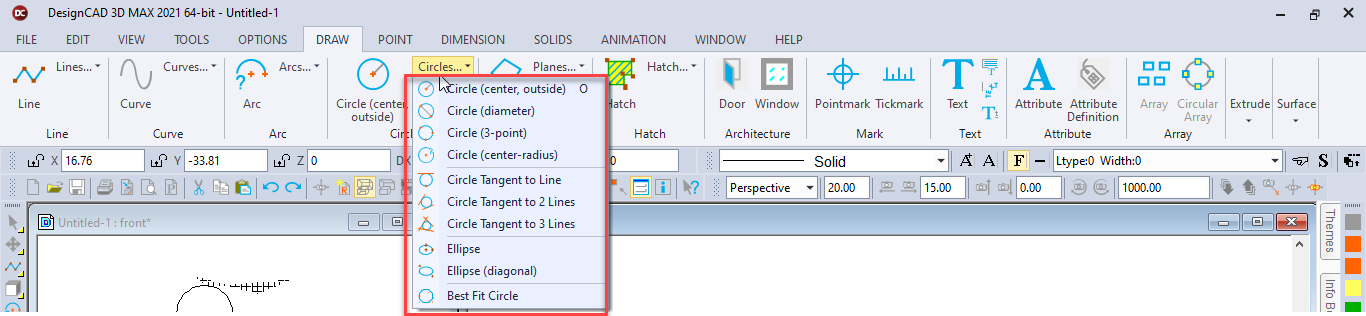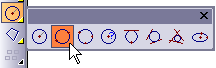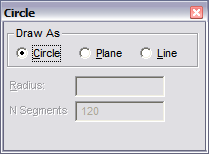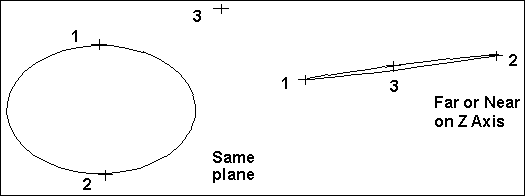# Circle (Diameter)Toolbox Icon:Draws a circle between two points, so that the two points lie on the diameter of the circle.

Point 1: A point on the diameter of the circle

Point 2: A point for the opposite end of the diameter

Point 3: A point to align the circle in 3D space (optional)

There are three choices for how you want to save the circle:Circle: Stored as an actual circle in the drawing.

Plane: Stored as a circular plane with 120 sides,plane circles can be shaded.

Line: Stored as a line entity.

Normally, you’ll want to save the circle in the Circle form. If you want to use the circle as you would a solid, select Plane. Save the circle as a Line if you want to be able to scale the circle or treat it as a line entity.

Set two points for the circle diameter. If necessary, you can set a third point to define the plane on which the circle lies: hold down Ctrl+Shift while moving the mouse forward or backward and notice how the circle swings on a hinge set by the first two points. When the circle lies in the plane you want it to, set the third point. If you just want to set two points, press Enter after you have set them.N Segments: When saved as a plane or line, this is the number of segments, into which the object will be divided.